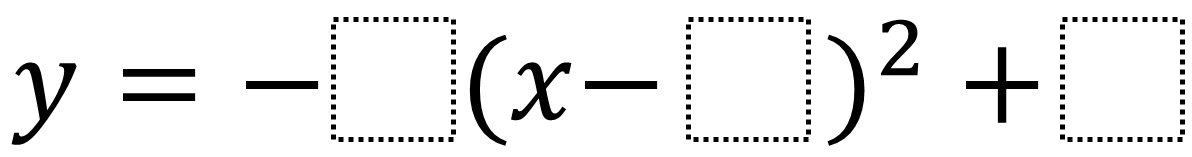# Quadratic In Vertex Form With Given Root And Maximum Value

Directions: Using the digits 1 to 9 at most one time each, place a digit in each box to create two different quadratic equations that have a root at 4 and a maximum value of 4. You may reuse all the digits for each equation.### Hint

What strategies can you use besides guessing and checking? What does having a maximum value of 4 tell you about the equation? What must x and y equal when there is a root at 4? How can using all of this information help you determine what a and h may equal?